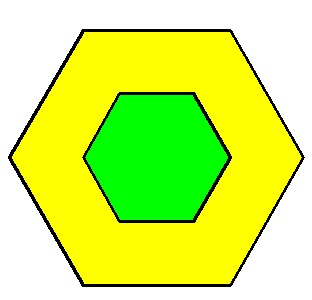# Ratio of areas to ratio of perimetersThe centers of the two regular hexagons shown below coincide.

The ratio of the area of the yellow region to the area of the green region is $3:1.$

What is the ratio of the perimeter of the big hexagon to the perimeter of the small hexagon?

×